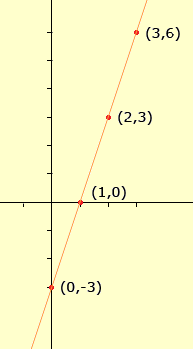MY DAUGHTER HAS A HOMEWORK PROLEM THAT I CAN NOT SOLVE: HERE ARE THE COORDINATED (0,-3), (1,0), (2,3),(3,6) WHAT IS THE EQUATION? Hi, I plotted the 4 points and noticed immediately that if you lie a ruler on the graph you can draw a straight line through all four points.The line is quite steep, in fact every time you increase the x-coordinate by 1 unit the y-coordinate increases by 3 units. It's almost like y = 3x, but that's not correct. Can you see how to adjust y = 3x to make it correct? Penny